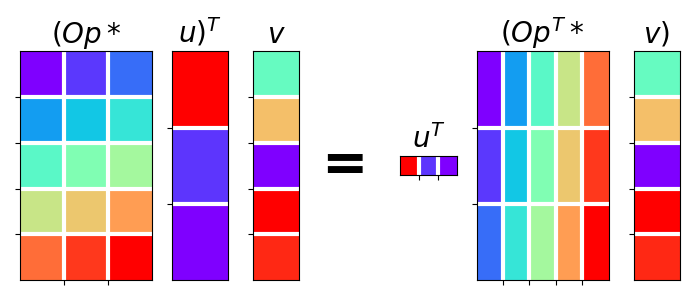# 02. The Dot-Test#

One of the most important aspect of writing a Linear operator is to be able to verify that the code implemented in forward mode and the code implemented in adjoint mode are effectively adjoint to each other. If this is the case, your Linear operator will successfully pass the so-called dot-test. Refer to the Notes section of `pylops.utils.dottest`) for a more detailed description.

In this example, I will show you how to use the dot-test for a variety of operator when model and data are either real or complex numbers.

```import matplotlib.gridspec as pltgs
import matplotlib.pyplot as plt

# pylint: disable=C0103
import numpy as np

import pylops
from pylops.utils import dottest

plt.close("all")
```

Let’s start with something very simple. We will make a `pylops.MatrixMult` operator and verify that its implementation passes the dot-test. For this time, we will do this step-by-step, replicating what happens in the `pylops.utils.dottest` routine.

```N, M = 5, 3
Mat = np.arange(N * M).reshape(N, M)
Op = pylops.MatrixMult(Mat)

v = np.random.randn(N)
u = np.random.randn(M)

# Op * u
y = Op.matvec(u)
# Op'* v
x = Op.rmatvec(v)

yy = np.dot(y, v)  # (Op  * u)' * v
xx = np.dot(u, x)  # u' * (Op' * v)

print(f"Dot-test {np.abs((yy - xx) / ((yy + xx + 1e-15) / 2)):.2e}")
```
```Dot-test 1.37e-16
```

And here is a visual intepretation of what a dot-test is

```gs = pltgs.GridSpec(1, 9)
fig = plt.figure(figsize=(7, 3))
ax = plt.subplot(gs[0, 0:2])
ax.imshow(Op.A, cmap="rainbow")
ax.set_title(r"\$(Op*\$", size=20, fontweight="bold")
ax.set_xticks(np.arange(M - 1) + 0.5)
ax.set_yticks(np.arange(N - 1) + 0.5)
ax.grid(linewidth=3, color="white")
ax.xaxis.set_ticklabels([])
ax.yaxis.set_ticklabels([])
ax.axis("tight")
ax = plt.subplot(gs[0, 2])
ax.imshow(u[:, np.newaxis], cmap="rainbow")
ax.set_title(r"\$u)^T\$", size=20, fontweight="bold")
ax.set_xticks([])
ax.set_yticks(np.arange(M - 1) + 0.5)
ax.grid(linewidth=3, color="white")
ax.xaxis.set_ticklabels([])
ax.yaxis.set_ticklabels([])
ax.axis("tight")
ax = plt.subplot(gs[0, 3])
ax.imshow(v[:, np.newaxis], cmap="rainbow")
ax.set_title(r"\$v\$", size=20, fontweight="bold")
ax.set_xticks([])
ax.set_yticks(np.arange(N - 1) + 0.5)
ax.grid(linewidth=3, color="white")
ax.xaxis.set_ticklabels([])
ax.yaxis.set_ticklabels([])
ax = plt.subplot(gs[0, 4])
ax.text(
0.35,
0.5,
"=",
horizontalalignment="center",
verticalalignment="center",
size=40,
fontweight="bold",
)
ax.axis("off")
ax = plt.subplot(gs[0, 5])
ax.imshow(u[:, np.newaxis].T, cmap="rainbow")
ax.set_title(r"\$u^T\$", size=20, fontweight="bold")
ax.set_xticks(np.arange(M - 1) + 0.5)
ax.set_yticks([])
ax.grid(linewidth=3, color="white")
ax.xaxis.set_ticklabels([])
ax.yaxis.set_ticklabels([])
ax = plt.subplot(gs[0, 6:8])
ax.imshow(Op.A.T, cmap="rainbow")
ax.set_title(r"\$(Op^T*\$", size=20, fontweight="bold")
ax.set_xticks(np.arange(N - 1) + 0.5)
ax.set_yticks(np.arange(M - 1) + 0.5)
ax.grid(linewidth=3, color="white")
ax.xaxis.set_ticklabels([])
ax.yaxis.set_ticklabels([])
ax.axis("tight")
ax = plt.subplot(gs[0, 8])
ax.imshow(v[:, np.newaxis], cmap="rainbow")
ax.set_title(r"\$v)\$", size=20, fontweight="bold")
ax.set_xticks([])
ax.set_yticks(np.arange(N - 1) + 0.5)
ax.grid(linewidth=3, color="white")
ax.xaxis.set_ticklabels([])
ax.yaxis.set_ticklabels([])
plt.tight_layout()
```From now on, we can simply use the `pylops.utils.dottest` implementation of the dot-test and pass the operator we would like to validate, its size in the model and data spaces and optionally the tolerance we will be accepting for the dot-test to be considered succesfull. Finally we need to specify if our data or/and model vectors contain complex numbers using the `complexflag` parameter. While the dot-test will return `True` when succesfull and `False` otherwise, we can also ask to print its outcome putting the `verb` parameters to `True`.

```N = 10
d = np.arange(N)
Dop = pylops.Diagonal(d)

_ = dottest(Dop, N, N, rtol=1e-6, complexflag=0, verb=True)
```
```Dot test passed, v^H(Opu)=-6.91965436475742 - u^H(Op^Hv)=-6.91965436475742
```

We move now to a more complicated operator, the `pylops.signalprocessing.FFT` operator. We use once again the `pylops.utils.dottest` to verify its implementation and since we are dealing with a transform that can be applied to both real and complex array, we try different combinations using the `complexflag` input.

```dt = 0.005
nt = 100
nfft = 2**10

FFTop = pylops.signalprocessing.FFT(
dims=(nt,), nfft=nfft, sampling=dt, dtype=np.complex128
)
dottest(FFTop, nfft, nt, complexflag=2, verb=True)
_ = dottest(FFTop, nfft, nt, complexflag=3, verb=True)
```
```Dot test passed, v^H(Opu)=(-17.13910350422714+3.8288339959112507j) - u^H(Op^Hv)=(-17.139103504227144+3.8288339959112494j)
Dot test passed, v^H(Opu)=(11.42080013111478-3.688151329246325j) - u^H(Op^Hv)=(11.420800131114781-3.6881513292463266j)
```

Total running time of the script: ( 0 minutes 0.323 seconds)

Gallery generated by Sphinx-Gallery